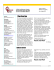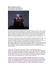# Parabolic Paths

## Transcription

Parabolic Paths
```Algebra 1
Embedded Assessment 1
Unit 5, page 453
Name ____________________________
Parabolic Paths
In 1680, Isaac Newton, scientist, astronomer, and mathematician, used a comet
visible from Earth to prove that some comets follow a parabolic path through
space as they travel around the sun. This and other discoveries like it help
scientists to predict past and future positions of comets.
1. Assume the path of a comet is given by the function 𝑦 = −𝑥 ! + 4.
a. Graph the path of the comet.
Explain how you graphed it.
b. Identify the vertex of the function.
c. Identify the maximum or minimum of the function.
d. Identify the domain and range.
e. Write the equation for the axis of symmetry.
2. Identify the table that represents a parabolic comet path. Explain why and
3. The graph at the right shows a portion of the path of a comet represented
by a function in the form 𝑦 = (𝑥 − ℎ)! + 𝑘.
a. Write the equation of the function.
b. Simplify your equation and rewrite in
standard form.
4. The equation and graph below represent two different quadratic functions
for the parabolic paths of comets. Identify the maximum of each function.
Which function has the greater maximum value?
Function 1:
Function 2:
𝑦 = −𝑥 ! + 4
* * * * * * * * * * * * * * * * * * * * * * * * * * * * * * * * * * * * * *
```

### SPACE ROCKS!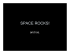### No. 68 OBSERVATIONS OF COMET IKEYA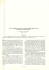### Bardot`s Comet -- Does Nature, Nurture, Numerology … or a Comet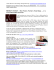### Document 6603749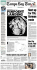### Parabolic Curve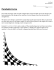### Comets, Meteors and Asteroids - 6th Grade Science with Mrs. Voris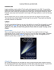### 1964 Mercury Cyclone.pptx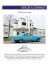### Halley`s Comet Strikes Back!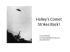### VOL 6.4